## 次方數學謎題

1) 如果 x = 1，則 y 是多少？
2) 如果 x = 2 ，則 y 又是多少？
3) 如果 y = 2 ，則 x 是多少？
4) 如果 y = 3 ，則 x 又是多少？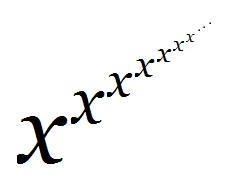註：注意這種連續次方，要從最上層的次方開始計算，一層次方算出的值作為下面一層的指數；也就是如上述 y 的式子中的括號所示。例如：21^3 = 21 = 2 ， 而不是 = (21)3 = 8 。

P.S. 以下內容是給 有算出第三題和第四題的朋友~

5) 如果 y = 4 ，則 x 又是多少？跟第三題的算出來的結果比較一下吧 ~

1) 1
2) 無限大
3) √2
4) 無解
5) 無解

(要證明的話，簡單來說，可以說明每算一層次方，數字至少變成原來的兩倍以上。既然 每次兩倍： 2, 4, 8, 16, ... 最後都會變成無限大，那 y = 2^(2^(2^(...^2))) 當然也是無限大。)

y = x^(x^(x^(x^(...^x)))) = x^(x^(x^(x^(x^(...^x))))) = x^y  = xy

3√3^(3√3^…^(3√3)) = 3
√2^(√2^…^(√2)) = 4 ，大於上一行的 3 。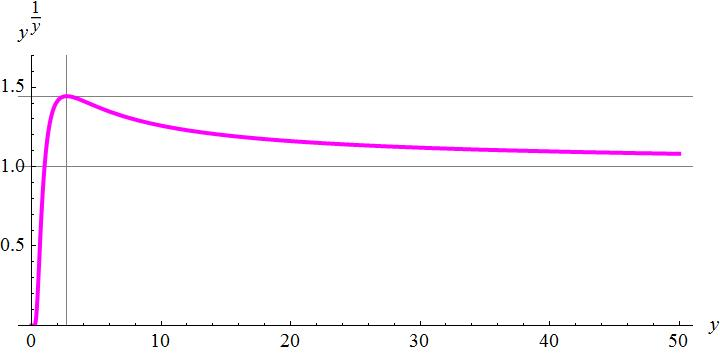• y = 1 的時候，y√y 也等於 1。
• y = 0 的地方 y√y 無定義，但是 y 趨近於 0 的時候，y√y 也趨近 0。
• y 趨近無限大的時候，y√y 趨近 1 (見上圖)。
• y = e ≒ 2.718 的時候，y√y 會有最大值 e√e ≒ 1.445 。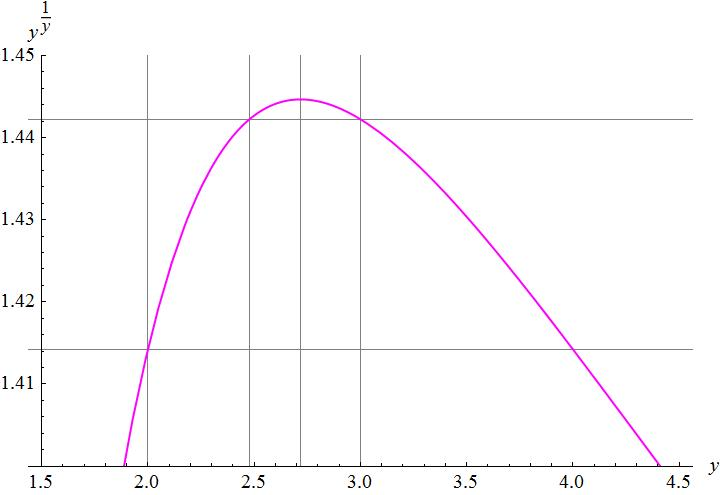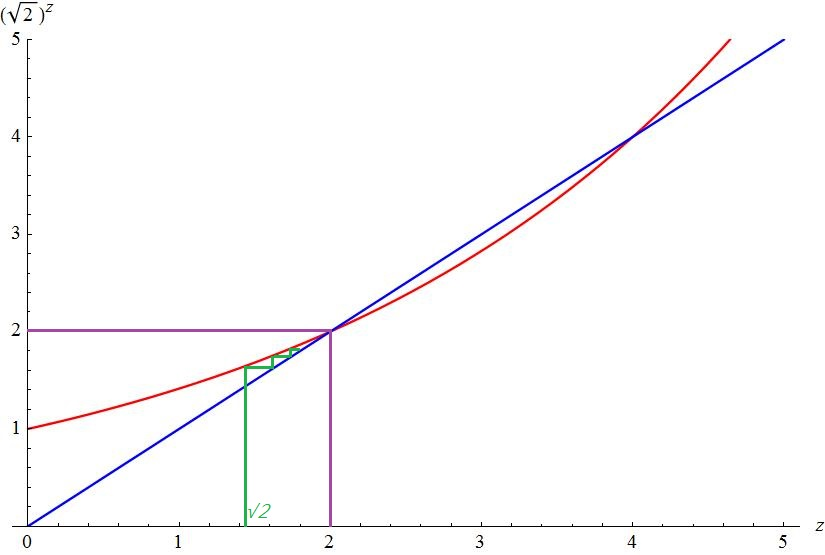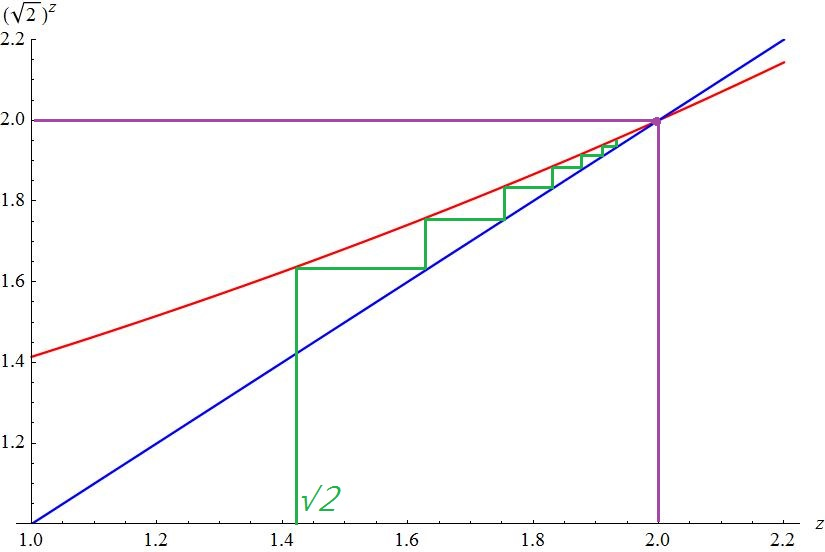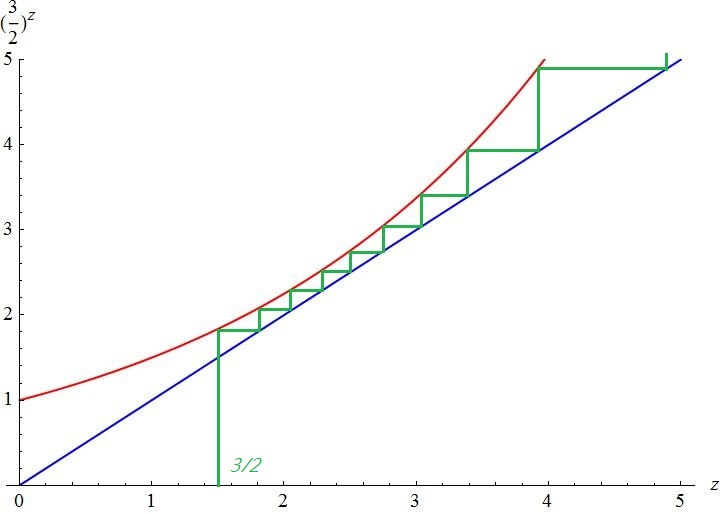• 當 y > e ≒ 2.718 的時候，y = x^(x^(x^(x^(...^x)))) 無法解出 x 來。
• 當 x > e√e  ≒ 1.445 時，y = x^(x^(x^(x^(...^x)))) 不存在 (會趨近無限大而發散)。

12,431